AJP Excel Information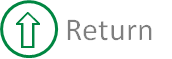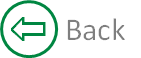##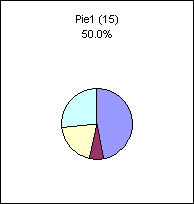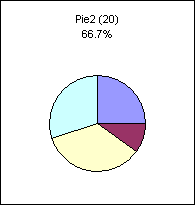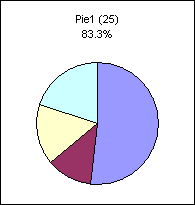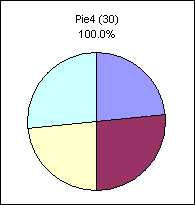##### Here is the data used to create the 4 separate pie charts.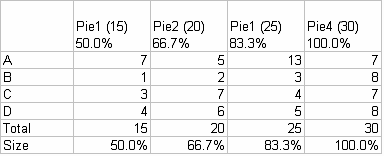##### Here are the formula used for Pie1. The other pies use similar formula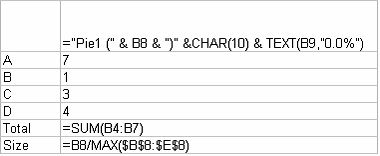##### The sizing is done via VBA code
 ```Sub SizePies() ' ' Resize pie charts plot area according to a ' Dim sngNormalSize As Single Dim objCht As ChartObject Dim rngSizes As Range Dim intIndex As Integer Dim sngTop As Single Dim sngWidth As Single ' size proportions of pies Set rngSizes = ActiveSheet.Range("B9:E9") Set objCht = ActiveSheet.ChartObjects(1) ' Using first chart as point of reference sngNormalSize = objCht.Chart.PlotArea.Width sngTop = objCht.Chart.PlotArea.Top ' go thru and resize each pie For Each objCht In ActiveSheet.ChartObjects With objCht.Chart intIndex = intIndex + 1 sngWidth = sngNormalSize * rngSizes.Cells(1, intIndex) If sngWidth > 10 Then .PlotArea.Width = sngWidth .PlotArea.Left = (.ChartArea.Width - .PlotArea.Width) / 2 .PlotArea.Top = sngTop + ((.ChartArea.Height - sngTop) - .PlotArea.Height) / 2 End If End With Next End Sub```
##### This workbook contains an all the formula, charts and VBA code.

Created August 2004
Last updated 5th August 2014

Microsoft® and Microsoft® Excel are registered trademarks of the Microsoft Corporation.
andypope.info is not associated with Microsoft. Copyright ©2007-2016 Andy Pope